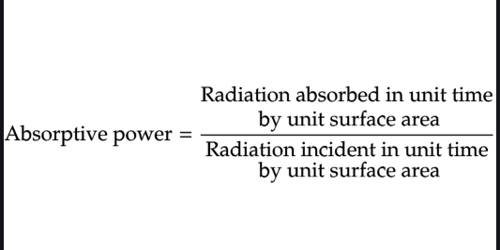# Define Absorptive Power

The absorptive power of a body (or a surface) is defined as the ratio of the energy absorbed in a given time (or in a certain time) to the radiant energy incident on it at the same instant of time. It is a measure of heat absorbed by an object. When heat is incident on the surface of the body some of the heat is absorbed while other is reflected.

Therefore absorptive power, a = Amount of energy absorbed / Amount of energy incident

When heat is incident on the surface an object, a part of it is absorbed and the remaining part is reflected. The absorptive power of a black body is 1 because it absorbs the radiant energy of all wavelength incidents on it. A good absorber is a good radiator. Usually, the absorbed radiation is converted to thermal energy, increasing the object’s temperature.  A Black body, for instance, absorbs all incident radiation, and its absorptive power is 1. So, the body which has higher absorptive power has a higher emissive power also. The amount of heat absorbed by the body depends upon the nature of the body.

Absorptive power of a body is defined at a given temperature and wavelength is defined as the ratio of the amount of heat energy absorbed to the amount of heat energy incident on it in a wavelength range. Scientists make use of this thermal energy for producing electricity. Absorption is the most proficient mode of converting low-grade heat into electricity.  There are other uses as well. The continuous-cycle absorption cooling unit is operated by the application of a limited amount of heat. This heat is furnished by gas, electricity, or kerosene.